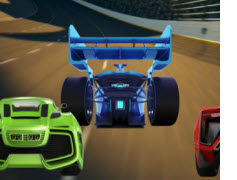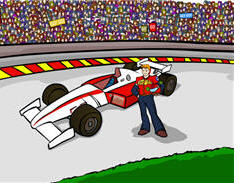# Math Racing Games

Do you love racing games? Try one of these cool math racing games and see how much fun you can have when you practice your math skills in an interactive way.

You will have a lot of fun when you play these fast-paced racing games! Check them out!Addition with Regrouping Math Racing Game

Play this exciting Math racing game about adddition with regrouping. Hairpin turns, bumper-to-bumper fast-paced action is all here.Compare Decimals Math Racing Game

In this exciting Math racing game, compare decimals in order to outlast the competition and cross the finish line first. Hairpin turns, bumper-to-bumper fast-paced action is all here.Order and Compare Numbers to 100

Math racing game about ordering and comparing two-digit whole numbers.

Place Value Game

In this place value game students will identify large numbers in standard and word form.

Multiplication Game
Car racing game about multiplying one-digit whole numbers.

Properties of Operations Math Racing Game
Experience the joy and excitement of online car racing as you identify the properties of equivalent expressions in this Properties of Operations Math Racing Game.

Give the sum of a number and its double in this fun Add Doubles Math Racing Game.

Balance the Addition Equation Math Racing Game
Quickly give the solution that makes both sides of the addition equation equal in this fun Balance the Addition Equation Math Racing Game.

Balance the Subtraction Equation Math Racing Game
Give the number that makes the subtraction equation correct in this fast-paced Balance the Subtraction Equation Math Racing Game.

Number Facts Math Racing Game

Make practicing the four operations fun by playing this exciting Number Facts Math Racing Game. But, you have to work fast, or your "racecar" won't make to the checkered flag.

Multiply within 100 Math Racing Game
In this great Multiply within 100 Math Racing Game, you can really enjoy multiplying numbers as you try to finish this fast-paced learning game.

Divide within 100 Math Racing Game
Quickly solve these division problems in this fun Divide within 100 Math Racing Game as your racecar screams around the racetrack to get to the finish line.

One-Step Equations Math Racing Game
Solve the one-step equations in order to compete in this fast-paced car racing game and cross the finish line first in this One-Step Equations Math Racing Game.

When playing this game, students will understand that repeated addition problems can be used to model multiplication problems.Round Decimals Math Racing Game

Quickly (but carefully) round these decimals to the given place value in order to cross the finish line first in this fast-paced Round Decimals Math Racing Game.